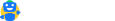accounting-and-auditing

# Liquidity Ratio, Formula With Examples

Updated on:

## Liquidity ratio

It’s a ratio that tells one’s ability to pay off its debt as and when they become due. In other words, we can say this ratio tells how quickly a company can convert its current assets into cash so that it can pay off its liability on a timely basis. Generally, Liquidity and short-term solvency are used together.

## The usefulness of liquidity ratio

The liquidity ratio affects the credibility of the company as well as the credit rating of the company. If there are continuous defaults in repayment of a short-term liability then this will lead to bankruptcy. Hence this ratio plays important role in the financial stability of any company and credit ratings.

## Formulas

Under liquidity ratio there are several more ratios, which come into the picture for checking how financially, sound a company is:

I. Current Ratio

II. Acid Test Ratio or Quick Ratio

III. Absolute Liquidity Ratio

IV. Basic Defense Ratio

### Current Ratio

This ratio measures the financial strength of the company. Generally, 2:1 is treated as the ideal ratio, but it depends on industry to industry.

Formula:  Current Assets/ Current Liability, where

A. Current Assets = Stock, debtor, cash and bank, receivables, loan and advances, and other current assets.

B. Current Liability = Creditor, short-term loan, bank overdraft, outstanding expenses, and other current liability.

### Acid Test Ratio or Quick Ratio

This ratio is the best measure of liquidity in the company. This ratio is more conservative than the current ratio. The quick asset is computed by adjusting current assets to eliminate those assets which are not in cash.

Generally, 1:1 is treated as an ideal ratio.

Formula: Quick Assets/ Current Liability, where,

Quick Assets = Current Assets – Inventory – Prepaid Expenses

### Absolute liquidity ratio

This ratio measures the total liquidity available to the company. This ratio only considers marketable securities and cash available to the company. This ratio only tests short-term liquidity in terms of cash, marketable securities, and current investment. Formula:  Cash + Marketable Securities / Current Liability

### Basic Defense Ratio

This ratio measures the no. of days a company can cover its Cash expenses without the help of additional financing from other sources.

Formula: (Cash + Receivables + Marketable Securities) ÷ (Operating expenses +Interest + Taxes) ÷ 365

### Example:

 Particulars Amount Cash and Cash Equivalent 2188 Short-Term Investment 65 Receivables 1072 Stock 8338 Other Current Assets 254 Total Current Assets 11917 Accounts Payable 4560 Outstanding Expenses 809 Taxes Payable 307 Deferred Revenue 998 Income Tax Payable 227 Other Outstanding Expenses 1134 Total Current Liability 8035

1. Operating Expenses during the year is 2188
2. Net Interest paid during the year is 25
3. Taxes paid/ for the year is 1913
4. Current Ratio = Current Assets/Current Liability                                                                   = 11971 ÷8035   = 1.48
5. Quick Ratio = (Current Assets- Inventory)/Current Liability                                         = (11971-8338)÷8035    = 0.45
6. Basic Defense Interval = (Cash + Receivables + Marketable            Securities) ÷ (Operating expenses +Interest + Taxes)÷365                                                                 = (2188+1072+65)÷(11215+25+1913)÷365                                                     = 92.27
7. Absolute liquidity ratio =(Cash + Marketable Securities)÷ Current Liability  =(2188+65) ÷ 8035                                                                                                 = 0.28Free, simple and accurate. Designed by tax expertsHave a query?
mailto@cleartax.in
Company
Individuals
Tax Experts
SMEs
Enterprises
ITR Resources
MF Resources
Tools
ClearGST
ClearServices